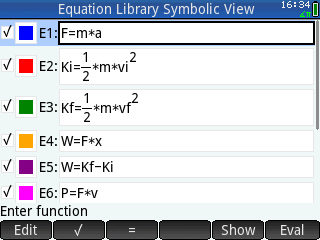SolveSys - Equation Library and Nonlinear Systems Solver

## Description

This is an app that implements a quasi-Newton solver (Newton-Raphson algorithm with backtracking linesearch). The app comes with a collection of systems of equations as found in the Equation Library on the HP48G series (and on the HP Solve application card for the HP48SX). Users may also create their own systems and save them for later use by adding on to the current library.

## Screenshots## Archive Contents

Name Size
icon.png 1.6 KB
install.txt 1.2 KB
Merged Version/Equation Library.hpappdir/Equation Library.hpapp 1.3 KB
Merged Version/Equation Library.hpappdir/Equation Library.hpappprgm 158.0 KB
Merged Version/Equation Library.hpappdir/Equation Library.hpappvars 116 bytes
Merged Version/Equation Library.hpappdir/Equation Library.lib 100.5 KB
Merged Version/Equation Library.hpappdir/icon.png 1.6 KB
Separated Version/Equation Library.hpappdir/Equation Library.hpapp 1.3 KB
Separated Version/Equation Library.hpappdir/Equation Library.hpappprgm 112.2 KB
Separated Version/Equation Library.hpappdir/Equation Library.hpappvars 116 bytes
Separated Version/Equation Library.hpappdir/Equation Library.lib 100.5 KB
Separated Version/Equation Library.hpappdir/icon.png 1.6 KB
Separated Version/SVD2.hpprgm 41.6 KB
Source/eqlib_lib.txt 94.1 KB
Source/equation_library.txt 91.4 KB
Source/equation_library_svd.txt 136.3 KB
Source/svd2.txt 21.3 KB
eqlib.pdf 182.3 KB
File Size
313.9 KB

Author
Han
6 years, 7 months ago

Rating
No ratings.